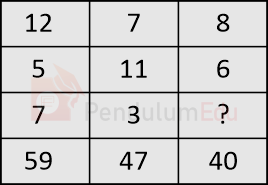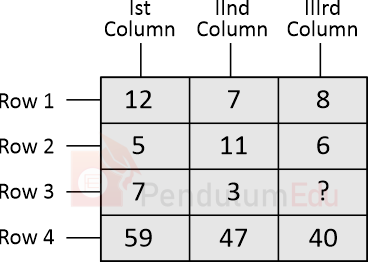Question of The Day04-03-2021

Find the missing term:Correct Answer : d ) 4

Explanation :The logic in the above table is column wise

The common logic in all the three columns is:

Term in row 4 = {[2 × (Term in row 1)] + [Term in row 2 × Term in row 3]}

Thus, considering Ist column

{[2 × 12] + [5 × 7]} = 24 + 35 = 59 (Term in row 4)

Considering IInd column

{[2 × 7] + [11 × 3]} = 14 + 33 = 47 (Term in row 4)

Considering IIIrd column

{[2 × 8] + [6 × ?]} = 40 (Term in row 4)

⇒ 16 + 6 × ? = 40

⇒ 6 × ? = 24

⇒ ? = Missing term = 4

Hence, (d) is the correct answer.

Such type of question is asked in various government exams like SSC CGL, SSC MTS, SSC CPO, SSC CHSL, RRB JE, RRB NTPC, RRB GROUP D, UPSC CDS, UPSC NDA, UP SI etc.

Read Daily Current Affairs, Banking Awareness, Hindi Current Affairs, Word of the Day, and attempt free mock tests at PendulumEdu and boost your preparation for the actual exam.0